# Chapter 2 Polynomials NCERT Exemplar Solutions Exercise 2.4 Class 10 Maths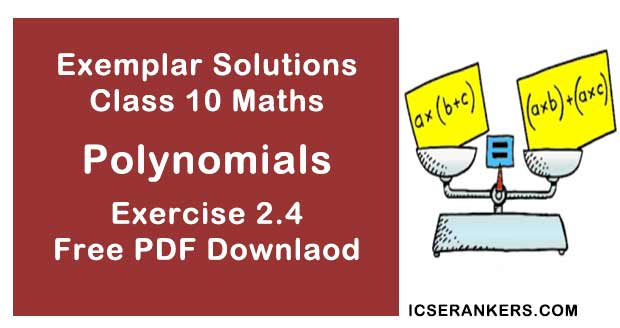Chapter Name NCERT Maths Exemplar Solutions for Chapter 2 Polynomials Exercise 2.4 Book Name NCERT Exemplar for Class 10 Maths Other Exercises Exercise 2.1Exercise 2.2Exercise 2.3 Related Study NCERT Solutions for Class 10 Maths

### Exercise 2.4 Solutions

1. 1. For each of the following, find a quadratic polynomial whose sum and product respectively of the zeroes are as given. Also find the zeroes of these polynomials by factorization.
(i) -8/3, 4/3
(ii) 21/8, 5/16
(iii) -2√3, -9
(iv) -3/2√5, -1/2

Solution

(i) Sum of the zeroes = -8/3
Product of the zeroes = 4/3
P(x) = x2 – (sum of the zeroes)x + (product of the zeroes)
So,
P(x) = x2 –x(-8/3) + 4/3
P(x) = 3x2 + 8x + 4
Splitting the middle term, we get,
3x2 - 8x + 4 = 0
⇒ 3x2 + 6x + 2x + 4 = 0
⇒ 3x(x + 2) + 2(x + 2) = 0
⇒ (x + 2)(3x + 2) = 0
⇒ x + 2 = 0
Or
3x + 2 = 0
⇒ x = -2, -2/3

(ii) Sum of the zeroes = 21/8
Product of the zeroes = 5/16
P(x) = x2 - (sum of the zeroes)x + (product of the zeroes)
P(x) = x2 - x(21/8) + 5/16
P(x) = 16x2 - 42x  + 5
Splitting the middle term,
16x2 - 42x + 5 = 0
⇒ 16x2 - (2x + 40x) + 5 = 0
⇒ 16x2 - 2x - 40x + 5 = 0
⇒ 2x(8x - 1) - 5(8x - 1) = 0
⇒ (8x - 1)(2x - 5) = 0
⇒ x = 1/5, 5/2

(iii) Sum of the zeroes = -2√3
Product of the zeroes = -9
P(x) = x2 - (sum of the zeroes)x + (product of the zeroes)
P(x) = x2 - 2√3x - 9
Splitting the middle term,
x2 - 2√3x - 9 = 0
⇒ x2 - (-√3x + 3√3x) - 9 = 0
⇒ x2 + √3x - 3√3 x - 9 = 0
⇒ x(x + √3) - 3√3 (x + √3) = 0
⇒ (x + √3)(x - 3√3) = 0
⇒ x = -√3, 3√3

(iv) Sum of the zeroes = -3/2√5
Product of the zeroes = -1/2
P(x) = x2 - (sum of the zeroes)x + (product of the zeroes)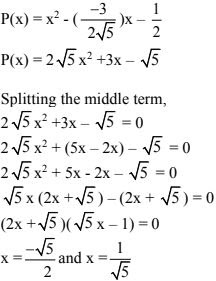2. Given that the zeroes of the cubic polynomial  x3 - 6x2 + 3x + 10 are of the form a, a + b, a + 2b for some real numbers a and b, find the values of a and b as well as the zeroes of the given polynomial.

Solution
We have, a, a+b, a+2b are roots of given polynomial x3 - 6x2 + 3x +10.
Sum of the roots = a+2b+a+a+b
= -coefficient of x2/coefficient of x3
3a + 3b = -(-6/1)
⇒ 3(a + b) = 6
⇒ a + b = 2
⇒ b = 2 - a
Product of roots = (a + 2b)(a + b)a
= - constant/coefficient of x3
(a + b + b)(a + b)a = -10/1
Putting the value of a + b =2, we get,
(2+b)(2) a = -10
⇒ (2+b) 2a = -10
⇒ (2+2-a) 2a = -10
⇒ (4-a)2a = -10
⇒ 4a - a2  = -5
⇒ a2 - 4a - 5 = 0
⇒ a2 - 5a + a - 5 = 0
⇒ (a - 5)(a + 1) = 0
⇒ a - 5 = 0 or a + 1 = 0
⇒ a = 5 and a = -1
Putting values of a in equation (i),
When a = 5,
5 + b = 2
⇒ b = -3
When a = -1
-1 + b = 2
⇒ b = 3

3. Given that √2 is a zero of the cubic polynomial 6x3 + √2 x2 - 10x - 4√2, find its other two zeroes.

Solution

According to question, √2 is one of the zero of the cubic polynomial.
So,
(x - √2) is one of the factor of the given polynomial,
P(x) = 6x3 + √2x2 - 10x - 4√2
So, by dividing p(x) by x - √2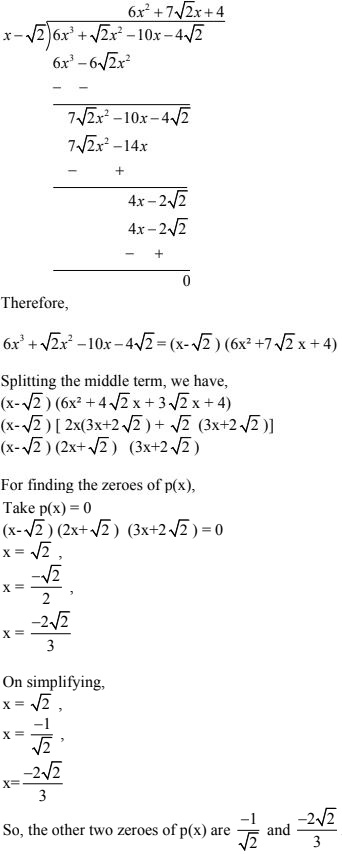4. Find k so that x2 + 2x + k is a factor of 2x4 + x3 - 14x2 + 5x + 6. Also find all the zeroes of the two polynomials.

Solution

By factor theorem and Euclid's division algorithm, we get
f(x) = g(x) × q(x) + r(x)
Taking,
f(x) = 2x4 + x3 - 14x2 + 5x + 6
and g(x) = x2 + 2x + k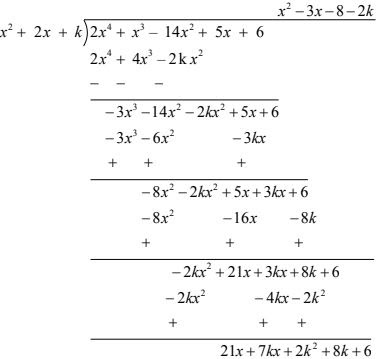But, r(x) = 0
(21 + 7k)x + 2k2 + 8k + 6 = 0x + 0
So,
21 + 7k = 0
⇒ k = -21/7
And
2k2 + 8k + 6 = 0
Splitting the middle term, we get,
2k2 + 6k + 2k + 6 = 0
⇒ 2k(k + 3) + 2(k + 3) = 0
⇒ (k + 3)(2k + 2) = 0
⇒ k + 3 = 0 or 2k + 2 = 0
Therefore, k = -3, -1.
Common solution is k = -3
q(x) 2x2 - 3x - 8 - 2(-3)
= 2x2 - 3x - 8 + 6
q(x) = 2x2 - 3x - 2
f(x) = g(x) q(x) + 0
= (x2 + 2x – 3) (2x2  – 3x – 2)
= (2x2  – 4x + 1x – 2) (x2 + 3x – 1x – 3)
= [2x(x – 2) + 1 (x – 2)][x(x + 3) – 1(x + 3)]
f(x) = (x - 2)(2x + 1)(x + 3)(x - 1)
For zeroes of f(x), f(x) = 0
(x - 1)(x - 2)(x + 3)(2x + 1) = 0
⇒ (x - 1) = 0,
⇒ (x - 2) = 0
⇒ (x + 3) = 0
and
2x + 1 = 0
⇒ x = 1,
⇒ x = 2,
⇒ x = -3 and x = -1/2
Hence, zeroes of f(x) are 1, 2, -3 and  -1/2. And, the zeroes of x2 +2x - 3 is 1, -3.

5. Given that x - √5 is a factor of the cubic polynomial x3 - 3√5x2 + 13x - 3√5, find all the zeroes of the polynomial.

Solution

Let f(x) = x3 - 3√5x2 + 13x - 3√5 and g(x) = x - √5
g(x) is a factor of f(x) so f(x) = q(x) x - √5.
On dividing f(x) by g(x) we get,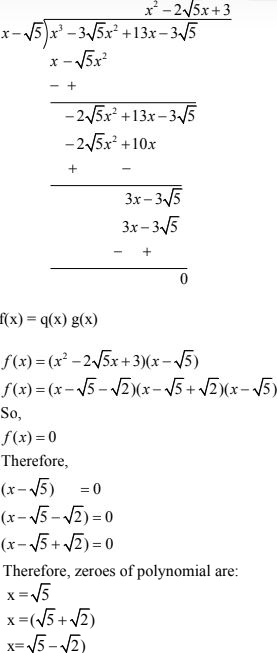6. For which values of a and b, are the zeroes of q(x) = x3 + 2x2 + a also the zeroes of the polynomial p(x) = x5 - x4 - 4x3 + 3x2  + 3x + b ? Which zeroes of p(x) are not the zeroes of q(x) ?
Solution
We have, factor theorem and Euclid's division lemma to solve this question,
Using factor theorem if q(x) is a factor of p(x), then r(x) must be zero.
p(x) = x5 - x4 - 4x3 + 3x2 + 3x + b
q(x) = x3 + 2x2 + a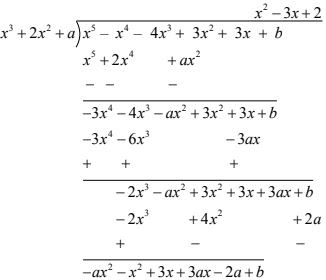So, by factor theorem remainder must be zero so,
r(x) = 0
-(a + 1)x2 + (3a + 3)x + (b - 2a) = 0x2 + 0x + 0
Comparing the coefficients of x2 , x and constant on both side, we get,
-(a + 1) = 0, 3a + 3 = 0 and b - 2a = 0
So,
a = -1 and
b - 2(-1) = 0
⇒ b = -2
For a = -1 and b = -2 zeroes of q(x) will be zeroes of p(x).
For zeroes of p(x),
p(x) = 0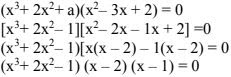So, x = 2 and 1 are not the zeroes of q(x).